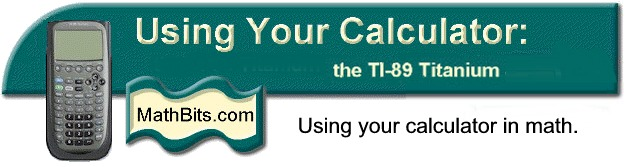Calculators for Upper Level High School and College Mathematics

 1.      •   Adjust the contrast   •    Find the HOME screen    •    Set the clock   •    Record ID number    •    Check out MODE key   •    Set the DEFAULTS   •    Turn off desktop APPS   •    Use the APPS key
 2.  •  Tabs from HOME screen •   Where's decimal answer? •   Expanding expressions •   Factoring polynomials •   Solving equations •   Finding zeros Hints: •   Editing •   Dealing w/unexpected ans •   Cleaning screen quickly •   Dealing  w/calc hang up
 •   Tabs from the Y= screen •   Set the window •   Evaluate specific values •   Defining functions

 •  Tabs Graph screen   •  Piecewise functions   •  Algebra of functions   •  Composition functions   •  Inverse functions

 5.   Limits   •  Limit command   •  Limits approach infinity   •  One-sided limits

 •  Differentiate command    •  Second derivative    •  Derivative at a value    •  Use of parameters    •  nDeriv command    •  nDeriv specific value    •  Formula for nDeriv

 •  Max/Min from HOME   •  Use of the "with" bar   •  Maximum from Graph   •  Minimum from Graph

 •   Integrate command    •   Find numerical values    •   Find antiderivative    •   Find antiderivative + C    •   nInt command

 9.   Other   •  Send/Receive          programs   (.pdf)   •  Send/Receive OS (.pdf)         (Operating System)